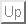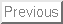\$B \$B\$^\$(\$,\$-(B   \$B:w0z(B

# [\$B%*!<%,%J%\$%:%I9V1i(B] \$B3NN(%b%G%k\$H=8CD:GE,2=F~Lg(B Introduction to statistical models for populational optimization

\$B@VJf(B \$B>1
Shotaro Akaho

### \$B35MW(B:

This paper provides an introductory review of populational search methods based on statistical modelling. We start with a random search algorithm called Markov Chain Monte Carlo (MCMC). To solve an optimization problem, it is crucial for the performance to use specific knowledge about the problem. We discuss how the optimizer can acquire the knowledge during the optimization, which is closely related to various fields of statistical learning theory.

Shotaro Akaho \$BJ?@.(B19\$BG/(B6\$B7n(B13\$BF|(B